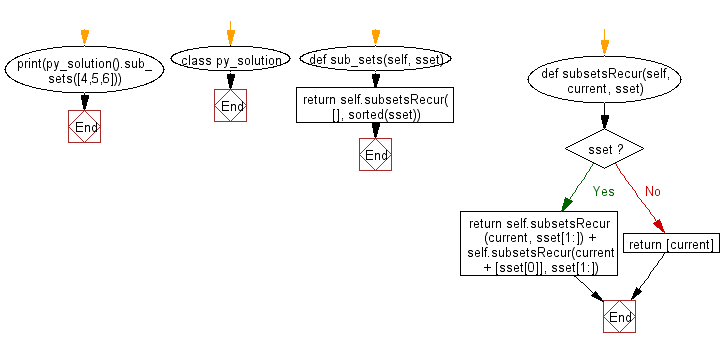﻿ Python: Get all possible unique subsets from a set of distinct integers - w3resource# Python: Get all possible unique subsets from a set of distinct integers

## Python Class: Exercise-4 with Solution

Write a Python program to get all possible unique subsets from a set of distinct integers.

Sample Solution:

Python Code:

``````class py_solution:
def sub_sets(self, sset):
return self.subsetsRecur([], sorted(sset))

def subsetsRecur(self, current, sset):
if sset:
return self.subsetsRecur(current, sset[1:]) + self.subsetsRecur(current + [sset], sset[1:])
return [current]

print(py_solution().sub_sets([4,5,6]))
```
```

Sample Output:

```[[], , , [5, 6], , [4, 6], [4, 5], [4, 5, 6]]
```

Pictorial Presentation:Flowchart:## Visualize Python code execution:

The following tool visualize what the computer is doing step-by-step as it executes the said program:

Python Code Editor:

What is the difficulty level of this exercise?

Test your Programming skills with w3resource's quiz.

﻿

## Python: Tips of the Day

The Zip() Function:

```>>> students = ('John', 'Mary', 'Mike')
>>> ages = (15, 17, 16)
>>> scores = (90, 88, 82, 17, 14)
>>> for student, age, score in zip(students, ages, scores):
...     print(f'{student}, age: {age}, score: {score}')
...
John, age: 15, score: 90
Mary, age: 17, score: 88
Mike, age: 16, score: 82
>>> zipped = zip(students, ages, scores)
>>> a, b, c = zip(*zipped)
>>> print(b)
(15, 17, 16)
```